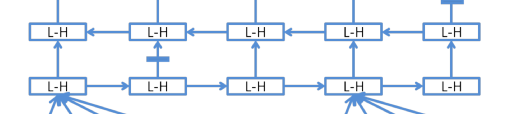# How to use a lstm in a reversed direction?

Hi, everyone! I am a newer on Pytorch. I have a question:
I want to build a BiLSTM which is different with the one provided by the Pytorch.
My idea BiLSTM is a forward LSTM from the left to right in a sentence. Then, each output of the forward LSTM hi will be feed into the reversed LSTM as the input. Just as the picture below shows.Question: Is there any way i can implement this process? Specially, when it comes to batching, it is a bit difficult to implement it.
Thanks!

If I don’t misunderstand, it seems to be straightforward to implement like:

``````h_f, _ = self.forward_lstm(x) # say h_f has size (batch, seq, dim)
inv_idx = torch.arange(h_f.size(1)-1, -1, -1).long()
inv_h_f = h_f.index_select(1, inv_idx)
h_b, _ = self.backward_lstm(inv_h_f)
``````

Then, you can manipulate `h_b` depending on your need. (be careful with the order as the input is reversed)